# Automatic Classification of Ovarian Cancer Types from CT Images Using Deep Semi-Supervised Generative Learning and Convolutional Neural Network

Automatic Classification of Ovarian Cancer Types from CT Images Using Deep Semi-Supervised Generative Learning and Convolutional Neural Network
Department of Computer Science, Sri Ramakrishna College of Arts and Science for Women, Coimbatore 641006, India

Corresponding Author Email:
honeypillaiphd@gmail.com
Page:
273-280
|
DOI:
https://doi.org/10.18280/ria.350401
18 June 2021
|
Accepted:
10 August 2021
|
Published:
31 August 2021
| Citation

OPEN ACCESS

Abstract:

The classification of ovarian cancer types is a very challenging process for physicians’ eyes. To solve this problem, this article proposes a new deep learner, which classifies ovarian cancer types from Computerized Tomography (CT) images. Firstly, a Deep Convolutional Neural Network (DCNN) model depending on AlexNet is proposed to categorize ovarian cancer from CT images. But its efficiency is not satisfactorily high. So, DCNN is built based on the fusion of AlexNet, VGG, and GoogLeNet. The fusion is carried out at the SoftMax layer by fusing the SoftMax values of each network structure using a weighted sum to obtain the overall classification outcome. But overfitting problems can occur due to an inadequate number of training images. Thus, a Deep Semi-Supervised Generative Learning with DCNN model (DSSGL-DCNN) is proposed by using a Generative Adversarial Network (GAN) which augments the training samples to solve the overfitting problem. Once the augmented dataset is obtained, the fused DCNN model is learned to classify ovarian cancer types. Further, the classified outcomes can be used as a useful guideline for physicians in medical diagnosis. Finally, the experimental results show that the DSSGL-DCNN achieves higher efficiency compared to the other DCNN architectures.

Keywords:

ovarian cancer, convolutional neural network, semi-supervised learning, generative adversarial network

1. Introduction

Among various kinds of cancer, ovarian cancer is the most widespread gynecologic malignancy. It accounts for 2.3% of all tumor mortality . It has the maximum death rate among all the gynecologic malignancies because most of the tumors are not diagnosed at an earlier stage. Effective chemotherapy applied to diagnose metastasized tumors is also crucial for enhancing the survival rate of ovarian cancer patients after surgery to remove major ovarian tumors. But a cancer diagnosis is a very complicated process that is vulnerable to human and training variation. Initially, a biopsy of abnormal cells is performed. After that, morphological and genetic analyses are executed. Such analyses are carried out on unstable scenarios to eliminate errors; however, false alarms still exist . The most reliable approach to decrease tumor mortality is to recognize it sooner. With the advancement of medical imaging, bioengineering studies have explored the use of different imaging modalities and deep learning models to assist the early detection provided by physicians .

Fusion of images captured from various imaging modalities and encouraging modern developments in imaging techniques which improve the accuracy of ovarian cancer classification in the area of Artificial Intelligence (AI) for computer vision purposes, where AI is combined with the imaging modalities to create Computer-Aided Diagnosis (CAD) systems . Recently, deep learning models using CT images have allowed the diagnosis to be highly efficient and also eliminate death rates and diagnostic delay. The key benefit of deep learning is the extraction of relevant knowledge from a vast quantity of data and its probabilities. Also, CT images have many advantages, such as broad availability, better reproducibility, highly cost-effective, and quick image scanning time. So, CT images are used in recent medicinal practice for ovarian cancer classification and diagnosis. Over the past few years, CNN has been widely developed to classify and diagnose different types of cancers, such as brain, liver, skin, etc., from CT images . But there is no effective classifier to categorize and diagnose ovarian cancer from CT images.

Therefore, this article initially proposes a DCNN model depending on AlexNet for categorizing ovarian cancer from CT images automatically. It encompasses 5 convolutional layers, 3 max-pooling layers and 2 reconnect layers. This model is learned from a given CT image dataset to categorize the variety of ovarian cancers. But its efficiency is not satisfied sufficiently. So, DCNN is built based on the fusion of three different structures, such as AlexNet, VGG, and GoogLeNet. Here, the results obtained from the last softmax layers of each network structure are fused by the weighted sum to obtain the ultimate classification outcome. Conversely, an overfitting problem can occur due to an inadequate number of training images. As a result, a DSSGL-DCNN model is proposed by using a GAN to solve the overfitting and data imbalance problems. The GAN is applied as an image augmentation method which increases the training samples for classification. Once the data augmentation is completed, the training image samples are fed to the DCNN based on fused architecture to train the model. After that, this trained model is used for categorizing the test samples into different categories of ovarian cancer. Thus, this DSSGL-DCNN based on fused architecture can enhance the accuracy of classifying ovarian cancer types from CT images.

Section 2 studies various deep learning models for classifying a variety of cancers. Section 3 explains the methodology of the DSSGL-DCNN model for ovarian cancer classification and Section 4 shows its efficiency. Section 5 summarizes the article and suggests future enhancements.

2. Literature Survey

Liao et al.  designed a new multi-task deep learning algorithm for classifying multiple cancers concurrently and enhancing the classifier efficiency of every cancer via leveraging the knowledge through shared layers. In this algorithm, the data across various processes was shared via configuring a shared hidden layer. It was constructed by considering 2 hidden layers and a softmax output layer. Also, the ReLU and sigmoid activation functions were selected in the hidden layers and the output layer, accordingly. It achieved a mean accuracy of 98.5%. However, it needs to classify more samples and enhance the classifier’s efficiency.

Burlina et al.  designed a DCNN model to predict the acute Lyme syndrome from erythema migrans images under varied circumstances. In this model, a cross-sectional image database was taken into consideration to learn the DCNN and categorize erythema migrans vs. tinea corporis, herpes zoster, and ordinary non-pathogenic skin. It had an accuracy of 86.53%, an AUC of 0.951 and a kappa of 0.7143. But its efficiency may be affected by classifying irrelevant images.

Hosny et al.  suggested an automated skin lesion categorization using transfer learning and pre-trained Deep Neural Network (DNN). Here, transfer learning was employed on the AlexNet in various modes: i) changing the architecture weights, ii) modifying the classification layer with a softmax, and iii) expanding the dataset through constant and random steps. The softmax was used for the classification of melanoma, seborrheic keratosis, and nevus. It achieved a mean accuracy of 95.91% for the ISIC dataset. On the other hand, it has less efficiency for larger quantities of images.

Li et al.  proposed pulmonary nodule recognition from thoracic MR images. In this technique, a faster Residual-CNN was designed by using the optimized parameters, a spatial 3-channel input structure, and transfer learning for finding the lung nodule regions. After that, a False Positive (FP) minimization method was proposed based on the anatomical features for minimizing the FPs and preserving the true nodules. It achieved a sensitivity of 85.2% with 3.47 false positives per image. But a few small and low contrast nodules were not identified. Also, a few artifacts and juxta heart tissues were wrongly identified as nodules.

Ozdemir et al.  proposed a novel end-to-end probabilistic diagnostic system using 3D DCNN for lung cancer detection and diagnosis. This system has two major modules, namely a CADe module and a CADx module. The CADe module was used for detecting and segmenting suspicious lung nodules, whereas the CADx module was applied for performing both nodule-level analysis and patient-level malignancy classification by analyzing the suspicious lesions from CADe. It attained a maximum AUC of 0.885, which indicates that the model and data uncertainties provide a valuable measure to identify patients. However, the efficiency was limited when the large nodule annotations were not enough.

Joyseeree et al.  suggested a new technique for classifying the different tags of infected and healthy pulmonary tissues from CT according to the concatenation of Riesz and deep features. Initially, discriminative parametric pulmonary tissue texture signs were trained from Riesz interpretations via a 1-vs.-1 method. The signs were created for different categories of tissues. Then, features from deep CNNs were computed by fine-tuning the inception V3 framework via augmented images. Finally, these trained interpretations were merged into a mutual softmax for classifying the lung tissues. It realized a mean accuracy of 74.4%, which was not highly effective.

Ge et al.  developed a novel pneumonia prediction framework for patients with acute ischaemic stroke using a different machine and deep learning algorithm. Initially, a dataset was collected which included a set of stroke patients with and without pneumonia. Then, the time-sensitive features were extracted and fed to the linear regression, SVM, Extreme Gradient Boosting (XGBoost), Multi-Layer Perceptron (MLP), and Recurrent Neural Network (RNN) for classifying pneumonia within different time windows. It achieved an AUC of 0.928 and 0.905 for pneumonia prediction within 7 days and 14 days, respectively. But it needs to categorize other types of diseases or cancers.

Dong et al.  recommended the Hybridized Fully CNN (HFCNN) to segment and predict liver cancer from CT images. During training, the CT images were collected and improved through data augmentation. Then, the features were extracted via training different layers of CNNs. During classification, a texture classifier was applied to differentiate usual and unusual hepatic lesions. Also, the abstract operations were used to separate Hepatocellular Carcinoma (HCC), liver cysts, and hemangiomas abnormal hepatic lesions for predicting liver cancer. It has a mean dice coefficient of 0.92 and has very precise liver volume measurements of 97.22%. But it considers only a limited amount of data to validate its efficiency.

Wu et al.  developed a DCNN fused with SVM to segment brain cancer images with the aid of three different processes. First, a DCNN was trained to learn the mapping from image space to cancer sign space. Then, the DCNN outcomes were given as input to the integrated SVM classifier with the testing images. Further, a DCNN and an integrated SVM were merged in sequence for training a deep classifier. It achieved a sensitivity of 92.4%, which was higher than the separate SVM and CNN. But its computation and processing time were high. Dutta et al.  designed an efficient CNN model for forecasting coronary artery syndrome. First, the Least Absolute Shrinkage and Selection Operator (LASSO)-based attribute weight estimation with majority voting was used to select the essential attributes. Then, these essential features were homogenized via a fully connected layer for forecasting coronary artery syndrome. Its balanced accuracy was 79.5%, but it has a high computational cost while increasing the number of neurons in each layer.

Yaar et al.  suggested a new DNN framework for forecasting chemo-sensitivity in ovarian cancer patients. It depends on Multiple Instance Learning (MIL) and an alternated Learning using Privileged Information (LUPI). LUPI was used to facilitate the data exchange of very useful privileged attributes which were obtainable only at the training phase. Also, this model was learned from the Hematoxylin and Eosin (H&E) stained multi-gigapixel Whole-Slide Images (WSIs) of ovarian cancer tissue patches and their related genomic expression data and the privileged attribute space. An improved generalization was achieved via cross-domain data exchange with a new mixture of MIL and LUPI. It has an average AUC-ROC of 0.8. But it considers a small dataset for evaluating the efficiency.

3. Proposed Methodology

In this section, the ovarian cancer classification from CT images using DCNN-AlexNet, DCNN-Fusion, and DSSGL-DCNN models is explained briefly. The key concept of this work is to use the ensemble deep learning classifier and classify ovarian tumor categories automatically. Due to the inadequate number of training samples, the learning of DCNN structures is not highly effective. So, by augmenting the training samples using DSSGL, the learning efficiency for DCNN structures is also increased, which results in high classification accuracy.

3.1 DCNN-AlexNet for ovarian cancer classification

DCNN is built based on AlexNet for automatically categorizing ovarian cancer CT images. The block diagram of the DCNN-AlexNet model for classifying ovarian cancer categories is depicted in Figure 1.

This structure comprises 5 convolutional, 3 max-pooling and 2 complete reconnect layers. Every layer is followed by the Rectified Linear Unit (ReLU) as the activation factor. Also, 3 max-pooling layers with a dimension of 3*3 pixels and stride 2 are applied to minimize the image dimension, which is the input of the next convolutional layer. Two fully connected layers comprising a huge number of neurons are applied at the end of DCNN. Since a fully connected layer engages most of the parameters, it is prone to overfitting. To solve the overfitting problem, a dropout strategy is employed and the dropout rate is 50%. The output is the likelihood determined by the SoftMax function for seven ovarian cancer categories, such as ovarian epithelial cancer, germ cell tumors, sex cord-stromal tumors, serous carcinoma, mucinous carcinoma, endometrioid carcinoma, and clear cell carcinoma.

Initially, the input image of dimension 227*227*3 is fed to the initial convolutional layer with 96 filters of 11*11 dimensions and consisting of similar padding and a stride of 4. The outcome of the initial layer is passed to the max-pooling layer (3*3) with a stride of 2 to reduce the feature map size, followed by the consecutive convolutional layers with 256, (5*5) filters and equal padding, i.e., the outcome height and width are retained as the prior layer, so the outcome from this layer is 27*27*256. The other convolutional function with 284, (3*3) filters consisting of equal padding is applied twice, providing the outcome of 13*13*384, followed by the other convolutional layer with 256, (3*3) filters and equal padding resulting in 6*6*256. Moreover, the flattening out is applied and 2 FC layers with 4096 neurons are further linked to the 7 unit SoftMax layer to categorize into 7 classes. This SoftMax layer gives the probabilities for every class to which an input image might belong.

## 1.pngFigure 1. AlexNet-based DCNN for ovarian cancer classification

3.2 DCNN-fused architecture for ovarian cancer classification

This new DCNN architecture is constructed by fusing three different structures: AlexNet, GoogLeNet, and VGG-19. The block diagram of DCNN based on fusion architecture for ovarian cancer classification is portrayed in Figure 2.

Initially, DCNN based on AlexNet structure is built in which 227*227 image is considered as the input. It is combined with 96 different kernels, every dimension 11*11 in the initial layer, and uses a greater stride of 4 pixels, which facilitates quick computation. The resulted 96 attribute maps of dimension 55*55 are initially conveyed via a ReLU and subsampled to 27*27 with a 3*3 max-pooling function. Finally, it is normalized through local input areas. Such processes are continued in layers 2, 3, 4, and 5. The final 3 layers are fully connected, considering every neuron in the prior layers as inputs and linking them to each neuron.

The FC6 and FC7 layers consist of 4096 neurons and a dropout likelihood of 0.5 is applied to avoid overfitting. The number of neurons in FC8 is identical to the number of labels. Finally, a softmax layer denotes the label ratings. This network is trained by a Stochastic Gradient Descent (SGD) with momentum. The batch size is assigned to 50 and the momentum is set to 0.9. Also, the multiplicative weight decay is allocated to 5×10-4 per epoch. The learning fraction begins at 0.001 and drops by a factor of 10 over the learning process, whereas the testing faults prevent the ongoing learning fraction.

Additionally, the DCNN based on the GoogLeNet structure is constructed, which has 22 layers. First, the input image dimension is 224*224 and then forwarded through 2 convolutional layers. The resulted attribute maps are applied to the sequence of inception units. The max-pooling is executed rather than FC at the top layer. The inception unit (i.e., I3, I4, and I5) is a mixture of many convolutional layers, and a parallel pooling with their output filters is integrated into the unified output vector for creating the input for the subsequent phase. In such layers, the kernel dimension is limited to 1*1, 3*3, and 5*5. This structure results in a significantly compact set of learning variables compared to the AlexNet. This network is trained by the SGD with a batch size of 8, momentum of 0.9, and weight decay of 2×10-4. The learning fraction begins at 0.001 and is reduced by a factor of 10.

## 2.png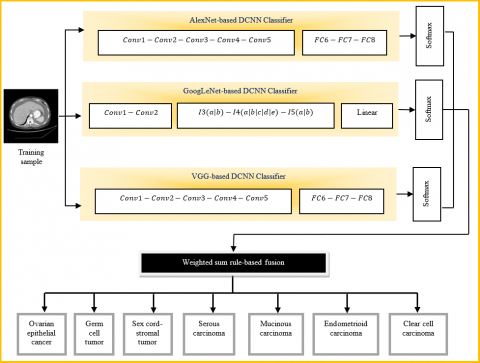Figure 2. Fusion architecture-based DCNN for ovarian cancer classification

## 3.png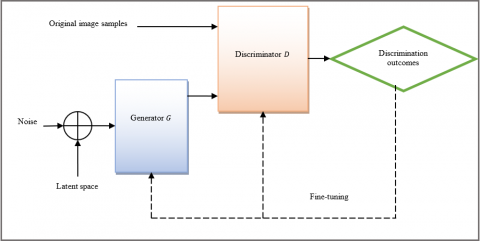Figure 3. Architecture of GAN-based data augmentatio

Similarly, DCNN based on the VGG-19 involves 5 convolutional and 3 FC layers. The input is a 224×224 image, and conv1 utilizes 7*7 kernels with stride 2. In conv3, conv4, and conv5, many kernels are employed compared to the AlexNet. The formation of FC layers is similar to AlexNet: the primary 2 layers consist of 4096 neurons each, whereas the third executes the ovarian cancer classification and consists of 7 outputs. Every hidden layer has a ReLU activation function and the softmax is the concluding layer. This network is trained by SGD with a batch size of 10 for optimizing the variables and a learning fraction of 10-4 combined with a momentum factor of 0.9. The learning is normalized via weight decay and the L2-penalty multiplier is assigned to 5 × 10-4.

Moreover, the classification accuracy is further enhanced by fusing the structures of AlexNet, GoogLeNet, and VGG. This concatenation is achieved at the last softmax layers and the outcomes obtained from each structure are fused by the weighted sum method for generating the resultant class. Here, the weights are assigned as 0.3, 0.4, and 0.3 for AlexNet, GoogLeNet, and VGG, respectively.

3.3 Deep semi-supervised generative learning with DCNN model for ovarian cancer classification

Although DCNN is based on the fusion of AlexNet, GoogLeNet, and VGG architectures, it considers a limited number of training samples, which still causes overfitting and training errors. Hence, the GAN is proposed with the DCNN model for augmenting the training samples and classifying the ovarian cancer categories. The GAN is applied in creating a synthetic image sample to augment the actual training sample while learning the DCNN model. GANs are built depending on adversarial nets for augmenting the image samples in an adversarial manner. The structure of GAN consists of 2 major networks: a generator G and a discriminator D. Here, G is used to map an image from a rectangular distribution to an image distribution, and D is learned to differentiate between actual and created images. In GANs, both G and D are trained simultaneously depending on the game premise. Figure 3 portrays the standard GAN structure for data augmentation.

In every iteration, G converts a noise vector Z quantized from a normal distribution $\left(X_{\text {forged }}=G(Z)\right)$ by a sequence of deconvolution and activation layers. Also, $D$ categorizes incoming images as genuine or forged. Typically, $D$ results the likelihood distribution over the sources $(S), P(S \mid X), S \in\{$ real, forged $\}$, via a sequence of convolutional and activation layers, increase the log-likelihood of the exact source $\left(L_{S}\right)$:

$L_{S}=\mathbb{E}\left[\log P\left(S=\operatorname{real} \mid X_{\text {real }}\right)\right]$$+\mathbb{E}\left[\log P\left(S=\right.\right. forged \left.\left.\mid X_{\text {forged }}\right)\right] (1) where, X_{\text {real }} is the real image, and X_{\text {forged }} is the forged image created by G . Then, G and D are learned in parallel by a minimax game with value function V(D, G) as: \min _{G} \max _{D} V(D, G)=\mathbb{E}_{X-P_{\text {data }}}[\log D(X)]$$+\mathbb{E}_{Z \sim \text { noise }}[\log (1-D(G(Z)))]$      (2)

In Eq. (2), $\mathbb{E}$ is the expectation operator, $D(X)$ is the chance of $X$ belonging to the actual data and $G(Z), X_{\text {forged }}$ is the sample created by $G$ from a random noise input $Z, X_{\text {real }}$ is the actual image sample from the dataset, and $P_{\text {data }}$ is the probability that $X$ came from the data rather than $P_{G}\left(P_{\text {data }}=\right.$ $\left.P_{G}\right)$.

The cross-entropy loss is used to compute the discriminator loss $\left(L_{D}\right)$ and the generator loss $\left(L_{G}\right)$ as follows:

$L_{D}=-\log D\left(X_{\text {real }}\right)-\log \left(1-D\left(X_{\text {forged }}\right)\right)$      (3)

$L_{G}=-\log D\left(X_{\text {forged }}\right)$      (4)

Normally, the discriminator is learned to distinguish whether the images generated by G are genuine or forged. In parallel, G is learned to generate images which are highly complex to be recognized by D as genuine or forged.

If the most favorable is attained, then G produces an image similar to the genuine image which can’t be distinguished by D. Through back-propagation, G trains the created image samples to highly resemble the training samples so that D can no longer differentiate them from the original samples. Based on the training of GAN, many training samples are generated for classification purposes, which solve the overfitting problem. Thus, the augmented training dataset is utilized to learn the fused DCNN model and classify it into 7 categories of ovarian cancer effectively. Thus, the DSSGL-DCNN based on the fused structure can be developed to classify ovarian cancer types by solving the overfitting problem.

Figure 4 shows the overall schematic representation of the proposed classification of ovarian cancer types.

## 4.png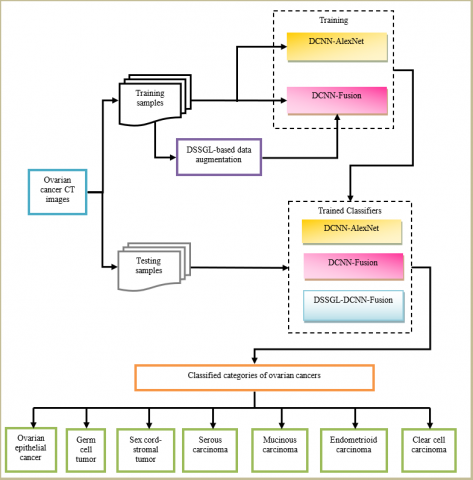Figure 4. Overall schematic representation of proposed ovarian cancer classification model

4. Experimental Results

In this section, different proposed DCNN models such as DCNN-AlexNet, DCNN-Fusion, and DSSGL-DCNN are implemented in MATLAB 2017b. This experiment is analyzed by gathering The Cancer Genome Atlas-Ovarian (TCGA-OV) dataset from cancer imaging that comprises the CT images in DICOM format. In this analysis, a total of 497 images are considered for class labels such as ovarian epithelial cancer, germ cell tumors, sex cord-stromal tumors, serous carcinoma, mucinous carcinoma, endometrioid carcinoma, and clear cell carcinoma. Among these, a total of 350 images are taken for training in which each class has 50 images. Similarly, a total of 147 images are taken for testing in which each class has 21 images. Also, the efficiency of these classifier models is compared with DNN , CADx , MLP , CNN , and MIL  in terms of precision, recall, f-measure, and accuracy.

4.1 Precision

It is the ratio of exactly classified categories of ovarian cancers at True Positive (TP) and False Positive (FP) rates.

Precision $=\frac{T P}{T P+F P}$      (5)

## 5.pngFigure 5. Comparison of precision

Figure 5 displays the results of precision achieved for DCNN-AlexNet, DCNN-Fusion, and DSSGL-DCNN models to classify the ovarian cancer types. From this analysis, it observes that the DSSGL-DCNN model based on fused architecture attains a higher precision than the DCNN-AlexNet and DCNN-Fusion models i.e., the precision of the DSSGL-DCNN (fusion) model is 33.29% increased than the MLP, 29.75% increased than the DNN, 22.51% increased than the CADx, 16.96% increased than the CNN, 15.28% increased than the MIL, 9.8% increased than the DCNN-AlexNet, and 4.85% increased than the DCNN-Fusion models.

4.2 Recall

It is the ratio of exactly classified categories of ovarian cancers at TP and False Negative (FN) rates.

Recall $=\frac{T P}{T P+F N}$      (6)

Figure 6 portrays the recall outcomes attained for DCNN-AlexNet, DCNN-Fusion, and DSSGL-DCNN models to categorize the ovarian cancers. This analysis indicates that the DSSGL-DCNN model depending on fused structure obtains a better recall than the DCNN-AlexNet and DCNN-Fusion models i.e., the recall of the DSSGL-DCNN (fusion) model is 33.7% higher than the MLP, 29.4% higher than the DNN, 22.3% higher than the CADx, 17.28% higher than the CNN, 15.58% higher than the MIL, 8.34% higher than the DCNN-AlexNet, and 3.43% higher than the DCNN-Fusion models.

## 6.png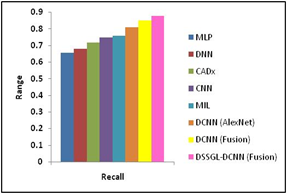Figure 6. Comparison of recall

4.3 F-measure

It is computed as the harmonic average of precision and recall.

$F-$ measure $=2 \times \frac{\text { Precision } \cdot \text { Recall }}{\text { Precision }+\text { Recall }}$      (7)

## 7.png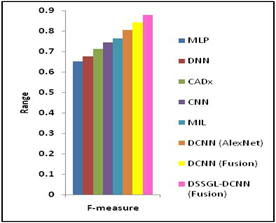Figure 7. Comparison of f-measure

Figure 7 shows the f-measure values for DCNN-AlexNet, DCNN-Fusion, and DSSGL-DCNN models for ovarian cancer classification. This analysis addresses that the DSSGL-DCNN based on combined networks provides a higher f-measure than the DCNN-AlexNet and DCNN-Fusion models i.e., the f-measure of DSSGL-DCNN (fusion) model is 33.9% greater than the MLP, 29.56% greater than the DNN, 22.51% greater than the CADx, 17.59% greater than the CNN, 14.82% greater than the MIL, 9.06% greater than the DCNN-AlexNet, and 4.14% greater than the DCNN-Fusion models.

4.4 Accuracy

It is the fraction of accurate classification of ovarian cancer categories over the total number of attempts executed.

Accuracy $=\frac{T P+\text { True Negative }(T N)}{T P+T N+F P+F N}$      (8)

TP is a result where DCNN classifiers categories the ovarian cancers as themselves e.g., clear cell carcinoma is classified as clear cell carcinoma. TN is a result where DCNN classifiers categories the non-ovarian cancers as non-ovarian cancers. FP is a result where DCNN classifiers inexactly categories ovarian cancers as non-ovarian cancers. FN is a result where DCNN classifiers inexactly categories the non-ovarian cancers as ovarian cancers.

## 8.pngFigure 8. Comparison of accuracy

Figure 8 demonstrates the accuracy values obtained by DCNN-AlexNet, DCNN-Fusion, and DSSGL-DCNN models for classifying the ovarian cancer types. By using this analysis, it is noticed that the DSSGL-DCNN model based on fused network structure achieves higher accuracy compared to the DCNN-AlexNet and DCNN-Fusion models i.e., the accuracy of the DSSGL-DCNN (fusion) model is 34.5% higher than the MLP, 29.6% higher than the DNN, 22.2% higher than the CADx, 17.6% greater than the CNN, 14.75% higher than the MIL, 9.33% higher than the DCNN-AlexNet, and 4.04% higher than the DCNN-Fusion models.

Additionally, the computational cost of DSSGL-DCNN (fusion) is measured as $O\left(\frac{1}{N^{4}}\right)+O(L B)$ where $N$ is the number of $\mathrm{CT}$ samples is the dataset, $L$ is number of layers used in DCNN, and $B$ is the batch size.

Thus, it is realized that the DSSGL-DCNN (fusion structure) has superior efficiency than the all other models. This is because of extending the number of training samples by using the DSSGL and classifying the CT samples using the fused structure-based DCNN. Therefore, the classification performance is increased significantly if learning more CT scans.

5. Conclusions

In this article, a DCNN model is initially designed based on AlexNet structure to categorize the ovarian cancer types from CT images. This DCNN is trained by the training dataset for classifying the types of ovarian cancers. However, its accuracy is not high. Therefore, DCNN is constructed depending on the fused structures of AlexNet, VGG, and GoogLeNet. The fusion is carried out at the last softmax layers of every structure and their softmax values are merged through a weighted sum for acquiring the final classification result.

But it learns only a limited amount of image samples which leads to an overfitting problem. To solve this problem, a DSSGL-DCNN model is developed in which the GAN is used to augment the training image samples. By using this augmented dataset, the DCNN based on fused architectures is trained and tested for categorizing the types of ovarian cancers. To conclude, the findings proved that the DSSGL-DCNN seems to have an accuracy of 87.84% compared to the DCNN based on AlexNet and fused structures for the different types of ovarian cancer classification.

References

 Nuhić, J., Spahić, L., Ćordić, S., Kevrić, J. (2019). Comparative study on different classification techniques for ovarian cancer detection. In International Conference on Medical and Biological Engineering, Springer, Cham, pp. 511-518. https://doi.org/10.1007/978-3-030-17971-7_76

 Lalremmawia, H., Tiwary, B.K. (2019). Identification of molecular biomarkers for ovarian cancer using computational approaches. Carcinogenesis, 40(6): 742-748. https://doi.org/10.1093/carcin/bgz025

 Forstner, R., Meissnitzer, M., Cunha, T.M. (2016). Update on imaging of ovarian cancer. Current Radiology Reports, 4(6): 1-11. https://doi.org/10.1007/s40134-016-0157-9

 Coccia, M. (2020). Deep learning technology for improving cancer care in society: New directions in cancer imaging driven by artificial intelligence. Technology in Society, 60: 101198. https://doi.org/10.1016/j.techsoc.2019.101198

 Xue, Y., Chen, S., Qin, J., Liu, Y., Huang, B., Chen, H. (2017). Application of deep learning in automated analysis of molecular images in cancer: A survey. Contrast Media & Molecular Imaging, 2017: 9512370. https://doi.org/10.1155/2017/9512370

 Liao, Q., Ding, Y., Jiang, Z.L., Wang, X., Zhang, C., Zhang, Q. (2019). Multi-task deep convolutional neural network for cancer diagnosis, Neurocomputing, 348: 66-73. https://doi.org/10.1016/j.neucom.2018.06.084

 Burlina, P.M., Joshi, N.J., Ng, E., Billings, S.D., Rebman, A.W., Aucott, J.N. (2019). Automated detection of erythema migrans and other confounding skin lesions via deep learning. Computers in Biology and Medicine, 105: 151-156. https://doi.org/10.1016/j.compbiomed.2018.12.007

 Hosny, K.M., Kassem, M.A., Foaud, M.M. (2019). Classification of skin lesions using transfer learning and augmentation with Alex-net. PloS One, 14(5): 1-17. https://doi.org/10.1371/journal.pone.0217293

 Li, Y., Zhang, L., Chen, H., Yang, N. (2019). Lung nodule detection with deep learning in 3D thoracic MR images. IEEE Access, 7: 37822-37832. https://doi.org/10.1109/ACCESS.2019.2905574

 Ozdemir, O., Russell, R.L., Berlin, A.A. (2019). A 3D probabilistic deep learning system for detection and diagnosis of lung cancer using low-dose CT scans. IEEE Transactions on Medical Imaging, 39(5): 1419-1429. https://doi.org/10.1109/TMI.2019.2947595

 Joyseeree, R., Otálora, S., Müller, H., Depeursinge, A. (2019). Fusing learned representations from Riesz filters and deep CNN for lung tissue classification. Medical Image Analysis, 56: 172-183. http://doi.org/10.1016/j.media.2019.06.006

 Ge, Y., Wang, Q., Wang, L., Wu, H., Peng, C., Wang, J., Yi, Y. (2019). Predicting post-stroke pneumonia using deep neural network approaches. International Journal of Medical Informatics, 132: 1-32. https://doi.org/10.1016/j.ijmedinf.2019.103986

 Dong, X., Zhou, Y., Wang, L., Peng, J., Lou, Y., Fan, Y. (2020). Liver cancer detection using hybridized fully convolutional neural network based on deep learning framework. IEEE Access, 8: 129889-129898. https://doi.org/10.1109/ACCESS.2020.3006362.

 Wu, W., Li, D., Du, J., Gao, X., Gu, W., Zhao, F., Yan, H. (2020). An intelligent diagnosis method of brain MRI tumor segmentation using deep convolutional neural network and SVM algorithm. Computational and Mathematical Methods in Medicine, 2020: 1-10. https://doi.org/10.1155/2020/6789306

 Dutta, A., Batabyal, T., Basu, M., Acton, S.T. (2020). An efficient convolutional neural network for coronary heart disease prediction. Expert Systems with Applications, 159: 113408. https://doi.org/10.1016/j.eswa.2020.113408

 Yaar, A., Asif, A., Raza, S.E.A., Rajpoot, N., Minhas, F. (2020). Cross-domain knowledge transfer for prediction of chemosensitivity in ovarian cancer patients. Proceedings of the IEEE/CVF Conference on Computer Vision and Pattern Recognition Workshops, pp. 4020-4025. https://doi.org/10.1109/CVPRW50498.2020.00472